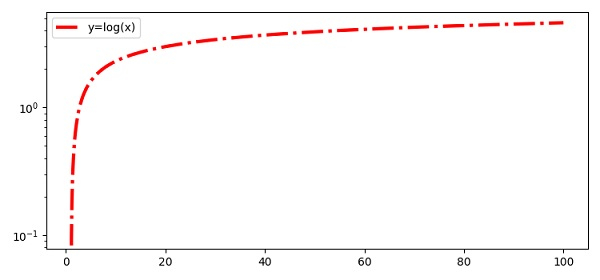# Logarithmic Y-axis bins in Python

To plot logarithmic Y-axis bins in Python, we can take the following steps −

• Create x and y points using numpy.

• Set the Y-axis scale using the yscale() method.

• Plot the x and y points, using the plot() method with linestyle="dashdot" and label="y=log(x)".

• To activate the label of the line, use the legend() method.

• To display the figure, use the show() method.

## Example

import numpy as np
from matplotlib import pyplot as plt
plt.rcParams["figure.figsize"] = [7.50, 3.50]
plt.rcParams["figure.autolayout"] = True
x = np.linspace(1, 100, 1000)
y = np.log(x)
plt.yscale('log')
plt.plot(x, y, c="red", lw=3, linestyle="dashdot", label="y=log(x)")
plt.legend()
plt.show()

## OutputUpdated on: 10-Apr-2021

2K+ Views